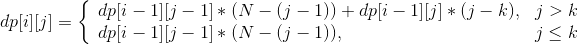0920. Number of Music Playlists

# 920. Number of Music Playlists#

## 题目 #

Your music player contains `N` different songs and she wants to listen to `L` ****(not necessarily different) songs during your trip. You create a playlist so that:

• Every song is played at least once
• A song can only be played again only if `K` other songs have been played

Return the number of possible playlists. As the answer can be very large, return it modulo `10^9 + 7`.

Example 1:

``````Input: N = 3, L = 3, K = 1
Output: 6
Explanation: There are 6 possible playlists. [1, 2, 3], [1, 3, 2], [2, 1, 3], [2, 3, 1], [3, 1, 2], [3, 2, 1].
``````

Example 2:

``````Input: N = 2, L = 3, K = 0
Output: 6
Explanation: There are 6 possible playlists. [1, 1, 2], [1, 2, 1], [2, 1, 1], [2, 2, 1], [2, 1, 2], [1, 2, 2]
``````

Example 3:

``````Input: N = 2, L = 3, K = 1
Output: 2
Explanation: There are 2 possible playlists. [1, 2, 1], [2, 1, 2]
``````

Note:

1. `0 <= K < N <= L <= 100`

## 题目大意 #

• 每首歌至少播放一次。
• 一首歌只有在其他 K 首歌播放完之后才能再次播放。

• 0 <= K < N <= L <= 100

## 解题思路 #

• 简化抽象一下题意，给 N 个数，要求从这 N 个数里面组成一个长度为 L 的序列，并且相同元素的间隔不能小于 K 个数。问总共有多少组组成方法。
• 一拿到题，会觉得这一题是三维 DP，因为存在 3 个变量，但是实际考虑一下，可以降一维。我们先不考虑 K 的限制，只考虑 N 和 L。定义 `dp[i][j]` 代表播放列表里面有 `i` 首歌，其中包含 `j` 首不同的歌曲，那么题目要求的最终解存在 `dp[L][N]` 中。考虑 `dp[i][j]` 的递归公式，音乐列表当前需要组成 `i` 首歌，有 2 种方式可以得到，由 `i - 1` 首歌的列表中添加一首列表中不存在的新歌曲，或者由 `i - 1` 首歌的列表中添加一首列表中已经存在的歌曲。即，`dp[i][j]` 可以由 `dp[i - 1][j - 1]` 得到，也可以由 `dp[i - 1][j]` 得到。如果是第一种情况，添加一首新歌，那么新歌有 N - ( j - 1 ) 首，如果是第二种情况，添加一首已经存在的歌，歌有 j 首，所以状态转移方程是 `dp[i][j] = dp[i - 1][j - 1] * ( N - ( j - 1 ) ) + dp[i - 1][j] * j` 。但是这个方程是在不考虑 K 的限制条件下得到的，距离满足题意还差一步。接下来需要考虑加入 K 这个限制条件以后，状态转移方程该如何推导。
• 如果是添加一首新歌，是不受 K 限制的，所以 `dp[i - 1][j - 1] * ( N - ( j - 1 ) )` 这里不需要变化。如果是添加一首存在的歌曲，这个时候就会受到 K 的限制了。如果当前播放列表里面的歌曲有 `j` 首，并且 `j > K`，那么选择歌曲只能从 `j - K` 里面选，因为不能选择 `j - 1``j - k` 的这些歌，选择了就不满足重复的歌之间间隔不能小于 `K` 的限制条件了。那 j ≤ K 呢？这个时候一首歌都不能选，因为歌曲数都没有超过 K，当然不能再选择重复的歌曲。(选择了就再次不满足重复的歌之间间隔不能小于 `K` 的限制条件了)。经过上述分析，可以得到最终的状态转移方程：• 上面的式子可以合并简化成下面这个式子：`dp[i][j] = dp[i - 1][j - 1]*(N - (j - 1)) + dp[i-1][j]*max(j-K, 0)`，递归初始值 `dp = 1`

## 代码 #

``````
package leetcode

func numMusicPlaylists(N int, L int, K int) int {
dp, mod := make([][]int, L+1), 1000000007
for i := 0; i < L+1; i++ {
dp[i] = make([]int, N+1)
}
dp = 1
for i := 1; i <= L; i++ {
for j := 1; j <= N; j++ {
dp[i][j] = (dp[i-1][j-1] * (N - (j - 1))) % mod
if j > K {
dp[i][j] = (dp[i][j] + (dp[i-1][j]*(j-K))%mod) % mod
}
}
}
return dp[L][N]
}

``````